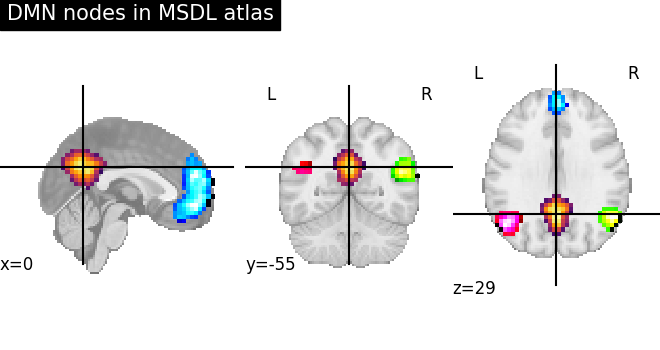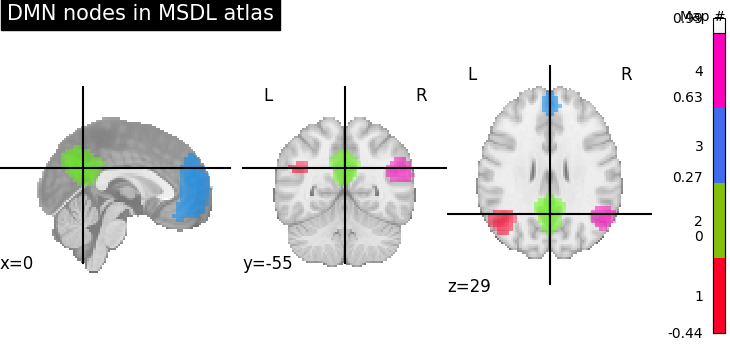# Visualizing a probabilistic atlas: the default mode in the MSDL atlas#

Visualizing a probabilistic atlas requires visualizing the different maps that compose it.

Here we represent the nodes constituting the default mode network in the MSDL atlas.

The tools that we need to leverage are:

Alternatively, `nilearn.plotting.plot_prob_atlas` allows to plot the maps in one step that with less control over the plot (see below)

## Fetching probabilistic atlas - MSDL atlas#

```from nilearn import datasets

atlas_data = datasets.fetch_atlas_msdl()
atlas_filename = atlas_data.maps
```
```Dataset created in /home/remi/nilearn_data/msdl_atlas

...done. (1 seconds, 0 min)
Extracting data from /home/remi/nilearn_data/msdl_atlas/8eaecb9e05c478f565847000d9902a25/MSDL_rois.zip..... done.
```

## Visualizing a probabilistic atlas with plot_stat_map and add_overlay object#

```from nilearn import image, plotting

# First plot the map for the PCC: index 4 in the atlas
display = plotting.plot_stat_map(
image.index_img(atlas_filename, 4),
colorbar=False,
title="DMN nodes in MSDL atlas",
)

# Now add as an overlay the maps for the ACC and the left and right
# parietal nodes
cmaps = [
plotting.cm.black_blue,
plotting.cm.black_green,
plotting.cm.black_pink,
]
for index, cmap in zip([5, 6, 3], cmaps):

plotting.show()
``````/home/remi/github/nilearn/env/lib/python3.11/site-packages/numpy/ma/core.py:2820: UserWarning: Warning: converting a masked element to nan.
_data = np.array(data, dtype=dtype, copy=copy,
```

## Visualizing a probabilistic atlas with plot_prob_atlas#

Alternatively, we can create a new 4D-image by selecting the 3rd, 4th, 5th and 6th (zero-based) probabilistic map from atlas via `nilearn.image.index_img` and use `nilearn.plotting.plot_prob_atlas` (added in version 0.2) to plot the selected nodes in one step.

Unlike `nilearn.plotting.plot_stat_map` this works with 4D images

```dmn_nodes = image.index_img(atlas_filename, [3, 4, 5, 6])
# Note that dmn_node is now a 4D image
print(dmn_nodes.shape)
```
```(40, 48, 35, 4)
```
```display = plotting.plot_prob_atlas(
dmn_nodes, cut_coords=(0, -55, 29), title="DMN nodes in MSDL atlas"
)
plotting.show()
```Total running time of the script: (0 minutes 6.246 seconds)

Estimated memory usage: 10 MB

Gallery generated by Sphinx-Gallery